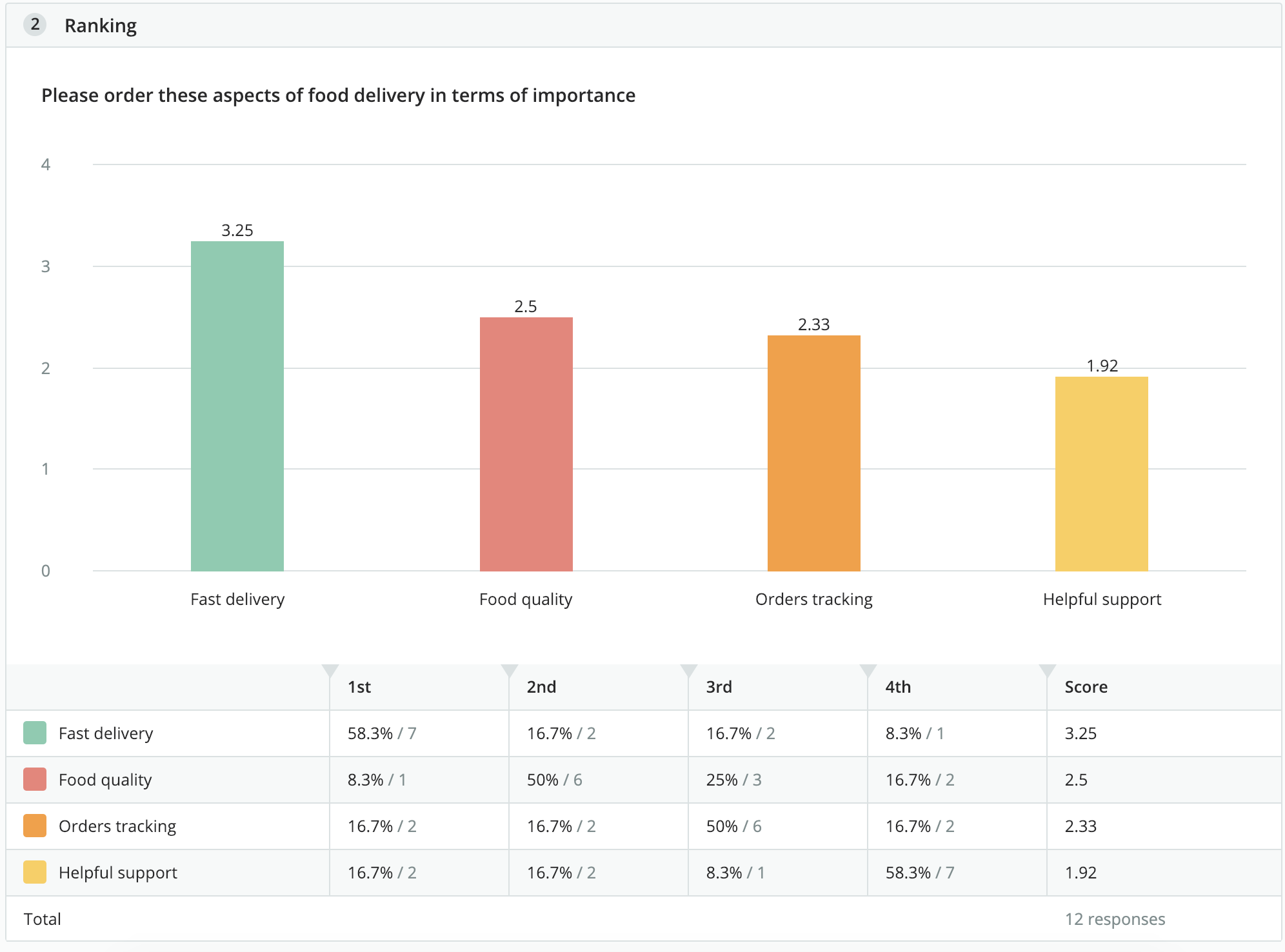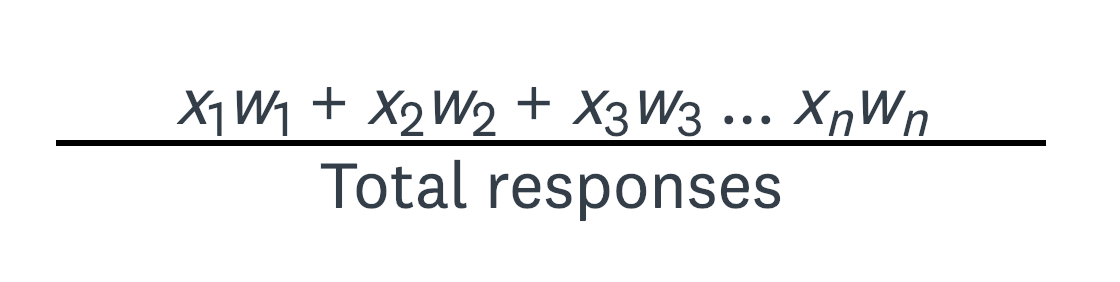How ranking question points are calculated?

The Ranking question asks respondents to compare items to each other by placing them in order of preference. In the Survey results section, an average ranking is calculated for each answer choice, allowing you to easily view and compare the overall preference for each item on the list.The average ranking is calculated using weighted average:where:

w = weight of ranked position
x = response count for answer choice

The respondent's most preferred choice (ranked as #1) has the largest weight, and their least preferred choice has a weight of 1.

For example, if a Ranking question has 5 choices to order, weights are assigned as follows:

• The #1 choice has a weight of 5
• The #2 choice has a weight of 4
• The #3 choice has a weight of 3
• The #4 choice has a weight of 2
• The #5 choice has a weight of 1